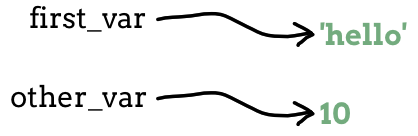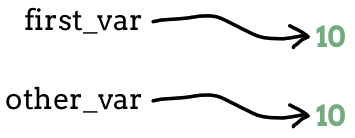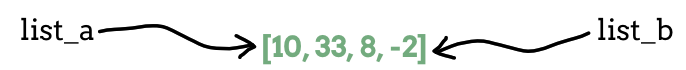# 8.7. Cloning Lists¶

Every data value that a program uses gets stored in a computer’s memory. When we assign a data value to a variable, that variable becomes a label for the memory location of that value.`first_var` points to `'hello'`, and `other_var` points to `10`.

What if we assign `first_var` and `other_var` the same value? For `first_var = 10` and `other_var = 10` each variable points to a different memory location, even though those locations store the same data.`first_var` points to `10`, and `other_var` points to a different `10`.

What if we assign one variable to another, like `other_var = first_var`? Does `other_var` point to a new memory location, or does it point to the same location as `first_var`?

Let’s perform a simple experiment to find out.

Example

Examine the output from the following program. How does the value of `other_var` change when `first_var` gets assigned a new value?

 ```1 2 3 4 5 6``` ```first_var = 10 other_var = first_var print(first_var, other_var) first_var = 'hello' print(first_var, other_var) ```

Console Output

```10 10
hello 10
```
1. Line 2 assigns the value of `first_var` to `other_var`.

2. Line 3 confirms that the two variables have the same value.

3. Line 5 assigns the new value `'hello'` to `first_var`.

4. Line 6 confirms that changing `first_var` does NOT affect `other_var`.

In this example, even though we set `other_var = first_var`, each variable points to a different location in memory. This allows us to replace `10` with `'hello'` for `first_var` without changing `other_var`.

Now let’s run the experiment again, but this time we will use a list as the data type.

Example

Examine the output from the following program.

 ```1 2 3 4 5 6 7 8 9``` ```list_a = [10, 33, 8, -2] list_b = list_a print(list_a, list_b) list_a.sort() print(list_a, list_b) list_b.append('hello') print(list_a, list_b) ```

Console Output

```[10, 33, 8, -2]   [10, 33, 8, -2]
[-2, 8, 10, 33]   [-2, 8, 10, 33]
[-2, 8, 10, 33, 'hello']   [-2, 8, 10, 33, 'hello']
```

The second set of results are quite different from the first. Any changes made with one variable affect the other!

Note

For the `int`, `str`, `bool`, and `float` data types, setting `other_var = first_var` creates separate values in two memory locations, one for each variable.

For lists, `list_b = list_a` creates two variables that point to the SAME memory location. The same list has two different names.

Because the same list has two different names, we say that it is aliased. Changes made with one alias affect the other.`list_a` and `list_b` both point to the same list.

## 8.7.1. Creating an Independent Copy¶

What if we want to modify a list but keep the original intact? In general, it is safer to avoid aliasing when working with lists or other mutable objects.

Making a separate copy of a list is called cloning, and Python gives us two ways to do it:

```list_clone = original_list.copy()
list_clone = original_list[ : ]
```

The `copy()` method creates a new list in a new memory location and assigns it to the `list_clone` variable. The empty slice operator `[ : ]` does the same thing, but it also allows us to clone a portion of the original list.

Try It!

Run the following program to confirm that, as written, changes made to either `list_a` or `list_b` affect the other.

1. In line 2, use the `copy()` method on `list_a`. Rerun the program to confirm that changes made to one list do NOT affect the other.

2. Repeat step 1, but use the slice operator instead of `copy()`.

Question

The syntax `list_a = list_b` creates a(n) ____.

1. alias
2. clone

Question

Changes made to a(n) ____ will NOT affect the original.

1. alias
2. clone

Question

What is printed by the following program?

 ```1 2 3 4``` ```list_a = [4, 2, 8, 6, 5] list_b = list_a list_b = 999 print(list_a) ```
1. [4, 2, 8, 6, 5]
2. [4, 2, 8, 999, 5]

Question

Describe a specific example or task where we would want to keep the original list intact.

Describe a specific example or task where it would not matter if we used an alias or a clone of the original list.